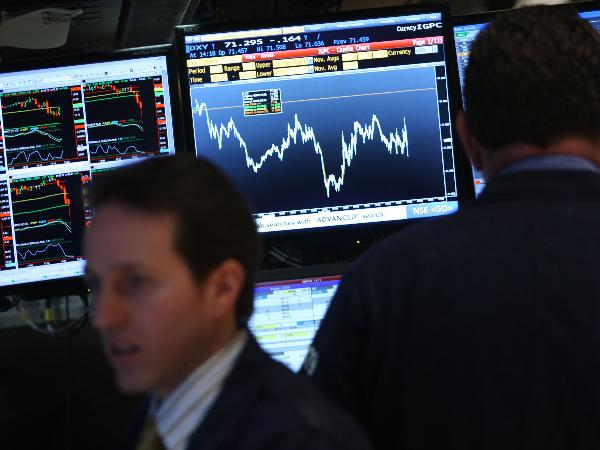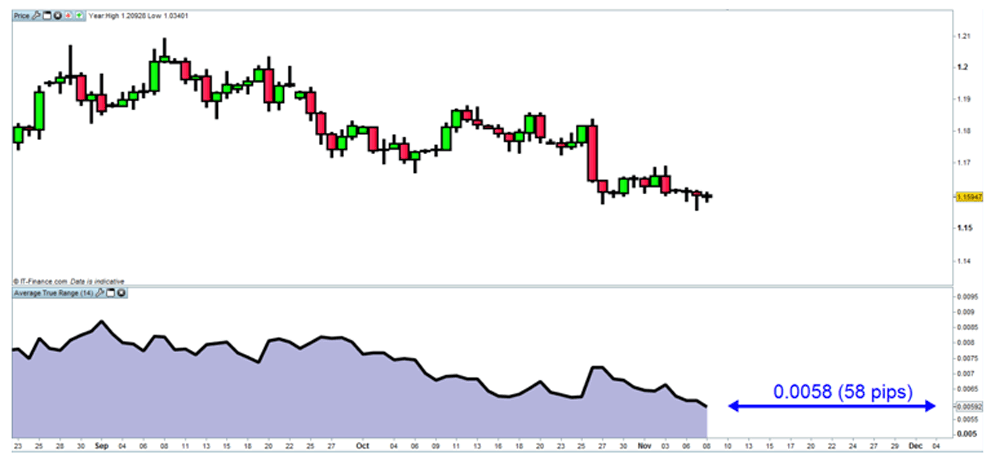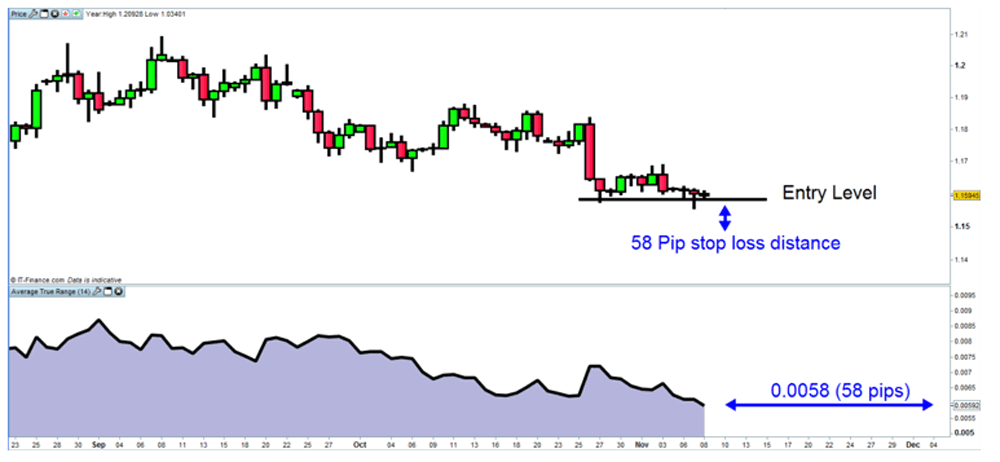# Effectively managing risk in the forex environment

Trading forex involves more risk than trading many other asset classes, and with that comes the potential for high reward or high loss. How you manage risk will, to a large extent, determine how successful you are at trading currency markets.Source: Bloomberg

The forex market is a high risk, potentially high reward, environment. If you have already made the decision to trade forex, you have made the decision to accept risk into your portfolio. Many traders will say, how you manage that risk will determine your level of trading success. Here, we look at one way a trader might effectively manage risk in the forex market by using a volatility stop loss methodology and position sizing technique.

### Volatility

There are a number of technical indicators that help measure market price volatility, including Bollinger bands, which use standard deviation calculations. But, for the following exercise, we are going to look at a simple price volatility indicator known as the average true range (ATR).

### EUR/USD chartThe above daily price chart is of EUR/USD, with the ATR indicator added. This indicator is measuring the average historical daily price range for the currency pair. The last reading of the ATR indicator on the chart is \$0.0058, which is equal to 58 pips (as one pip is four decimal places to the right on EUR/USD). So the ATR gives us the suggestion that 58 pips is a probable distance for the EUR/USD to move, over the course of a normal day.

The same principles or application of the ATR could be applied on varying time interval charts, not just the daily chart as in this example. On a five minute chart, the ATR indicator would give us a suggestion of the price movement distance over a five minute period. While a one hour ATR would suggest a possible price movement distance over an hour, and so on.

How does this help us?

### Volatility Stop Loss

Although the ATR gives us an expectation of how many points the EUR/USD currency pair might move in a day, it does not give us the expected market direction. However, by knowing how much a security might move over a period (over a day in this example) we are given an understanding of the possible risk. In the EUR/USD example, we now know that the pair could move 58 pips in a day. If I entered a long trade on EUR/USD, and it turned out to be a poor entry, my worst case scenario is expected to be a 58 pip move against me. In monetary terms, my contract size would determine my possible loss. So at \$1 a pip, I would lose \$58 (\$1 x 58 pips), and at \$10 a pip I would lose \$580 (\$10 x 58 pips).

One suggested use for the ATR is using the value (or a multiple thereof) as a stop loss distance from a trade entry point. The chart below, again of the EUR/USD, illustrates this idea.

### EUR/USD chartThe suggestion of using the ATR as a stop loss distance from our entry point is an attempt at trying to give a trade enough breathing room by considering the inherent price volatility in its calculation. In theory we would have to time our market entry as poorly as possible to be stopped out of the trade on a normal day. It could still happen, but should happen relatively infrequently.

The distance from our trade entry point to our protective stop level shows us how many pips we are risking in any one trade. We need to convert this into a currency value. A standard contract with IG on the EUR/USD would mean that one pip = \$10, while a mini contract with IG on EUR/USD would mean that one pip = \$1. For the sake of easy mathematics, we will use a mini contract for the following exercise.

So if our stop distance (using ATR as above) is 58 pips and we are trading in mini contract lot sizes then:

58 pips x \$1 pip = \$58

So for every \$1 mini EUR/USD contract we take, we would be risking \$58 on this trade.

### Position sizing

How many contracts a trader might consider trading can be referred to as our position size. A simple formula for this is as follows:

Position Size = Total Risk / Risk per contract (dollar terms)

We have defined the 'Risk per contract' in the previous section (Stop loss distance x value per contract/pip).

The 'Total Risk' refers to how much of your trading account you are prepared to risk in any one trade. Recommended guidelines would suggest not risking more than 2% to 5% of your trading capital, but this is personal to each trader. We recommend putting this Total Risk value into a currency amount. For example:

If Total Risk = 2% of account and account value is worth \$10,000

Then Total Risk = \$200

With this in mind, we can now calculate how many contracts to trade:

Position size = Total Risk / Risk Per Contract (dollar terms)

= \$200 / \$58

= 3.44

= 3 (rounded off)

So, to summarize as per the formula above, if we were to trade 3.44 mini EUR/USD contracts, with a stop distance of 58 pips, we would be risking \$200 on our actual trade.

We did however round down our contract size to three, which would mean we would actually be risking slightly less in our trade (\$58 x 3 = \$174).

### Conclusion

The above exercises are an attempt to help forex traders effectively manage their trading risk. We are using the average true range indicator to assess market price volatility. We are using this price volatility as an indicator to determine a stop distance, which has been converted into a dollar amount. By then establishing the total amount of our capital we are prepared to risk in any one trade, we can arrive at a position size for our trade by using this formula: Position size = Total Risk / Risk Per Contract (dollar terms).